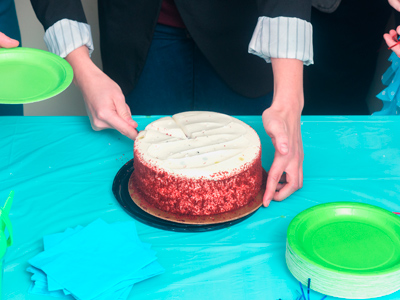Even numbers can be shared equally by two people, without having to cut them in half!

# Odd or Even

This Math quiz is called 'Odd or Even' and it has been written by teachers to help you if you are studying the subject at elementary school. Playing educational quizzes is a fun way to learn if you are in the 1st or 2nd grade - aged 6 to 8.

It costs only \$12.50 per month to play this quiz and over 3,500 others that help you with your school work. You can subscribe on the page at Join Us

Recognizing odd or even numbers is an important early number skill. It is one of the first properties of number children will be taught to recognize. Knowing odd and even will help children in other areas of their math development, including dividing numbers and completing sequences.

1.
Odd numbers end with the digits:
1, 2, 3, 4 or 5
1, 3, 5, 7 or 9
0, 1, 2, 4 or 6
2, 4, 6, 8 or 0
The last digit of an odd number is always one of these
2.
Even numbers always end with:
2, 3, 4, 5 or 6
1, 3, 5, 7 or 9
1, 2, 3, 4 or 5
2, 4, 6, 8 or 0
The last digit of an even number is always one of these
3.
Which of the following numbers is odd?
17
16
14
12
The last digit is a 7, which makes the number odd
4.
Which of the following sets of numbers are all odd?
2, 3, 4
10, 20, 30
11, 17, 21
12, 13, 14
All of these numbers end with an odd digit
5.
Which of these numbers is even?
17
18
15
13
The last digit is an 8, which makes this number even
6.
Which of the following sets of numbers are all even?
12, 13, 15
20, 22, 24
2, 4, 7
21, 23, 25
The last digit in each of these numbers is even, making the number even
7.
Which statement is true?
Even numbers can all be divided exactly by 2
Even numbers are always more than 20
Even numbers sometimes end with '3'
Even numbers always end with 2
Even numbers can be divided by two to give a whole number as the answer
8.
Which statement is true?
Odd numbers are all in the two times table
Odd numbers can end with a 2 or 4
Odd numbers are always bigger than even numbers
The last digit in an odd number is always 1, 3, 5, 7 or 9
Odd numbers always end with one of these digits, no matter how big they are
9.
Which set has an odd number in it?
2, 4, 6, 8, 10
2, 3, 4, 6, 8
6, 8, 10, 12, 14
10, 20, 30, 40, 50
3 is an odd number
10.
Which set has an even number in it?
1, 3, 5, 7, 9
31, 33, 35, 37, 39
23, 25, 27, 29, 31
13, 14, 15, 17, 19
14 is an even number
Author:  Angela Smith